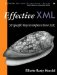# Java, C, Python, and Perl

### Java, C#, Python, and Perl

None of the common schema languages are Turing complete. Thus none of them can state and verify every possible constraint on an XML document. For example, none of them can verify that the content of a Prime element is in fact a prime number. However, this verification is straightforward in any reasonably powerful programming language. For example, here's a very na ve algorithm implemented in Java that accomplishes this task by dividing the number by every integer between 2 and its square root until it finds a divisor.

` public void isPrime(String text) {   int test;   try {     test = Integer.parseInt(text);   }   catch (NumberFormatException ex) {     return false;   }   if (test < 2) return false;   for (int i = 2; i < Math.sqrt(test); i++) {     if (test % i == 0) return false;   }   return true; } `

(Mathematically inclined readers should feel free to substitute a more efficient algorithm.)

For a slightly less mathematical example, consider an order form that can include multiple products.

` <Order>   <Product SKU="78X" quantity="12" price="21.95">     Clown Hat   </Product>   <Product SKU="83X" quantity="1" price="144.95" discount=".10">      Clown Car   </Product>   <Tax rate="7.0">27.57</Tax>   <Shipping  method="USPS" currency='USD'>8.95</Shipping>   <Total>430.37</Total> </Order> `

Before accepting the order, you want to multiply each quantity by its corresponding price, apply the discount to the items with discounts , calculate and add in the tax and shipping, and verify that it all equals the value of the Total element. In a traditional programming language, this is a CS101 problem. For example, using JDOM, a method might act like this:

` boolean orderValid(Element order) {     try {         Iterator products           = order.getChildren("Product").iterator();         double subtotal = 0;         while (products.hasNext()) {             Element product = (Element) products.next();             double price = Double.parseDouble(               product.getAttributeValue("price"));             int quantity = Integer.parseInt(               product.getAttributeValue("quantity"));             subtotal += price*quantity;         }         Element tax = order.getChild("Tax");         double rate = Double.parseDouble(           tax.getAttributeValue("rate"))/100.0;         if (Math.abs(subtotal * rate           - Double.parseDouble(tax.getText())) >= 0.01) {             return false;         }         subtotal += rate;         Element shipping = order.getChild("Shipping");         subtotal += Double.parseDouble(shipping.getText());         Element total = order.getChild("Total");         if (Math.abs(subtotal           - Double.parseDouble(total.getText()))>=0.01) {             return false;         }    }    catch (Exception ex) {      return false;    }    return true; } `

However, in the common schema languages, this problem is unsolvable . (RELAX NG actually allows you to plug in new data type libraries written in a traditional language like Java, Python, or C. The RELAX NG language itself can't verify these constraints without extra code like that shown here, but it does make it easier to insert your own custom code into the validation process.)

Traditional programming languages are also useful for verifying constraints that require access to external data. For example, you might want to look up the price of each item in an order in a remote database to verify that the process submitting the order hasn't gotten the price wrong. All major programming languages have libraries for talking to SQL databases. No schema languages do.Effective XML: 50 Specific Ways to Improve Your XML
ISBN: 0321150406
EAN: 2147483647
Year: 2002
Pages: 144